Home > MC2 > Chapter 11 > Lesson 11.3.1 > Problem11-82

11-82.
1. The two triangles below are similar. Homework Help ✎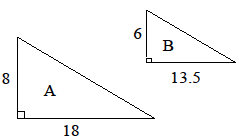1. Find the area of each triangle.

2. What is the scale factor for the side lengths of the two triangles?

3. What is the ratio of the areas (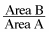)? How is this ratio related to the ratio you found in part (b)?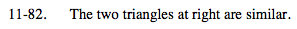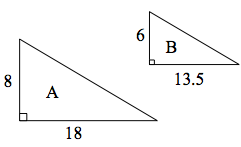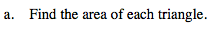$\frac{1}{2} (base)(height)$

Area A = 72 un2
Area B = 40.5 un2Find the ratio between one of the pairs of
corresponding sides for the two triangles.

$\text{Use \frac{6}{8} or \frac{13.5}{18}}.$

What do you get after simplifying?

$\frac{3}{4}$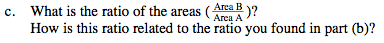How can you change the scale
factor to get this area ratio?

$\frac{40.5}{72} = \frac{9}{16}$